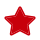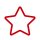# 案例：选择合适的分布列

#### 现象描述

 ```1 2``` ```CREATE TABLE t1 (a int, b int); CREATE TABLE t2 (a int, b int); ```

 `1` ```SELECT * FROM t1, t2 WHERE t1.a = t2.b; ```

#### 优化分析

 ```1 2``` ```CREATE TABLE t1 (a int, b int) DISTRIBUTE BY HASH (a); CREATE TABLE t2 (a int, b int) DISTRIBUTE BY HASH (a); ``````1 2``` ```CREATE TABLE t1 (a int, b int) DISTRIBUTE BY HASH (a); CREATE TABLE t2 (a int, b int) DISTRIBUTE BY HASH (b); ```# 相关产品